Next: Orbital parameters Up: Keplerian orbits Previous: Kepler's first law

# Kepler's third law

We have seen that the radius vector connecting our planet to the origin sweeps out area at the constant rate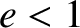. [See Equation (4.24).] We have also seen that the planetary orbit is an ellipse. Now, the major and minor radii of such an ellipse are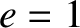and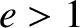, respectively. [See Equations (A.107) and (A.108).] The area of the ellipse is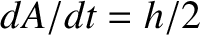. We expect the radius vector to sweep out the whole area of the ellipse in a single orbital period,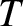. Hence,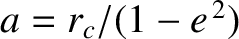(4.32)

It follows from Equation (4.31) that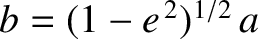(4.33)

In other words, the square of the orbital period of our planet is proportional to the cube of its orbital major radius--this is Kepler's third law of planetary motion.

Richard Fitzpatrick 2016-03-31